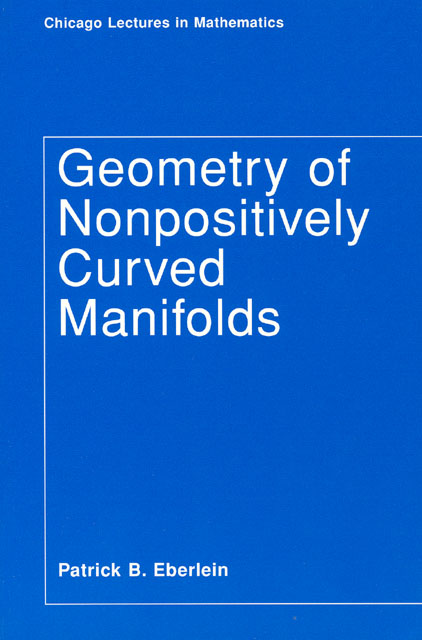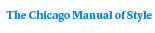# Geometry of Nonpositively Curved Manifolds

Patrick B. Eberlein456 pages | 6 x 9 | © 1997
Paper \$144.00 ISBN: 9780226181981 Published April 1997
Cloth \$153.00 ISBN: 9780226181974 Published April 1997
Starting from the foundations, the author presents an almost entirely
self-contained treatment of differentiable spaces of nonpositive
curvature, focusing on the symmetric spaces in which every geodesic lies
in a flat Euclidean space of dimension at least two. The book builds to
a discussion of the Mostow Rigidity Theorem and its generalizations, and
concludes by exploring the relationship in nonpositively curved spaces
between geometric and algebraic properties of the fundamental group.

This introduction to the geometry of symmetric spaces of non-compact
type will serve as an excellent guide for graduate students new to the
material, and will also be a useful reference text for mathematicians
Table of Contents• Contents
Contents
Acknowledgments
Introduction
1. Notation and Preliminaries
2. Structure of Symmetric Spaces of Noncompact Type
3. Tits Geometries
4. Action of Isometrics on M(infinity)
5. A Splitting Criterion
6. Isometries of R"
7. Spaces with Euclidean Factors
8. Mostow Rigidity Theorem
9. Rigidity Theorems and Characterizations of Symmetric Spaces of Higher Rank
10. Fundamental Group and Geometry
References
Index of Definitions and Terminology
Index of Notation